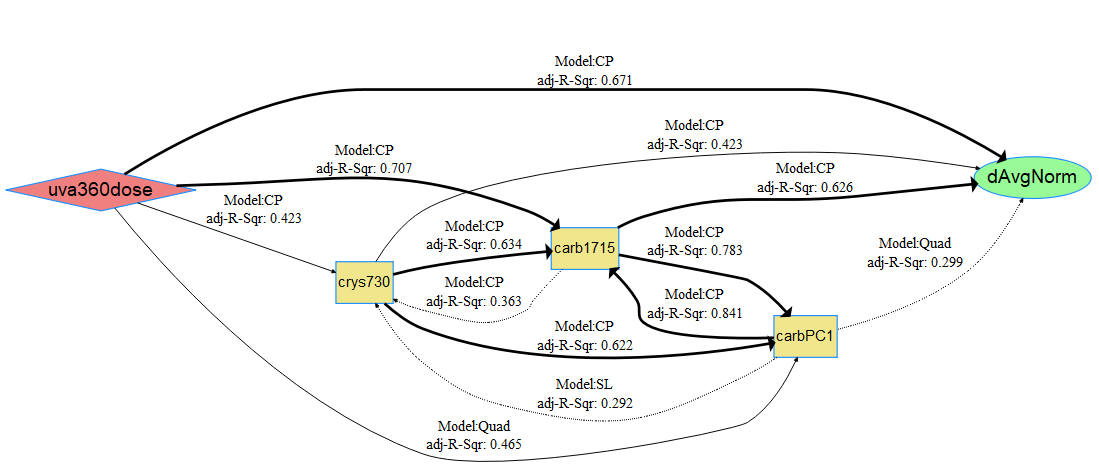Quantifying and Modeling Photovoltaic Backsheet Cracking

Data Description

A dataset of cracks in photovoltaic backsheets with inner layers of either ethylene-vinyl acetate or polyethylene exposed to 4,000 hours of continuous UVA irradiance with an intensity of 1.55 $$w/m^2$$ at 340 $$nm$$ and a chamber temperature of 70$$^\circ$$C. See the associated journal article in Polymer Degradation and Stability for details on obtaining quantitative crack measurements (Klinke et al. 2018).

130 observations of 5 variables are included:

• Response - dAvgNorm: average crack depth normalized by the backsheet’s inner layer thickness, dimensionless.
• Stress - uva360dose: cumulative, integrated irradiance dose received by the sample for all wavelengths $$<$$ 360 $$nm$$, in $$MJ/m^2$$.
• Mechanism - crys730: percent crystallinity of polyethylene regions determined from the ratio of Fourier transform infrared (FTIR) spectroscopy peak intensities at 731 and 720 $$cm^{-1}$$ (Huang, Hong, and Urban 1992), dimensionless.
• Mechanism - carb1715: ketone carbonyl index determined from the ratio of FTIR peak intensities at 1715 and 2851 $$cm^{-1}$$, dimensionless.
• Mechanism - carbPC1: 1st principal component score for the carbonyl region between 1500 and 1800 $$cm^{-1}$$.

Load data and run code to build netSEM

## Load the crack data set and preview column metadata
data(crack)
?crack

## Run netSEM
ans <- netSEMm(crack)

## Subset dataset with three cutoffs
res <- subsetData(ans, cutoff=c(0.4, 0.5, 0.6))

## Plot the network model
plot(ans, res)

Network diagram for data

dAvgNorm is the endogenous and all other variables are considered as exagenous. Based on the plot, the strongest pathway appears to be uva360dose $$\rightarrow$$ carb1715 $$\rightarrow$$ dAvgNorm. This can be confirmed using the pathwayRMSE() function.